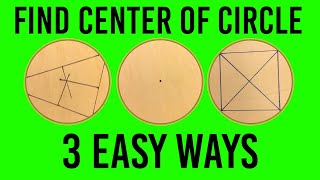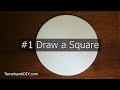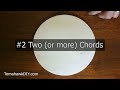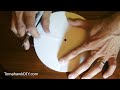# Find the Center of a Circle (3 EASY and QUICK Ways)

614K+ views   |   6K+ likes   |   661 dislikes   |
Jul 18, 2017

### Thumbs### Transcription

• - So you're trying to find the center of a circle?
• I'm gonna show you my three favorite ways to do that.
• I'm Mike.
• Welcome to TomahawkDIY.
• Few years ago, I made a video
• that got hundreds of thousands of views
• as people tried to figure out
• how to find the center of a circle.
• There were lots of great comments.
• I went through and tested all those different comments
• to see what I liked best.
• Came up with this.
• Take a look.
• I'm trying the circle method number one.
• So thanks to Oz Skes, Skies, Oz Skies,
• S-K-E-S, thanks to Oz for this method.
• I quite like this one.
• So Oz recommends drawing a square.
• So to draw a square, you wanna have a square.
• I'm gonna get a point here, and then we've gotta
• get our same length on each of these two sides.
• So let's see, four and a half,
• not quite, we're getting close though.
• It can be a little bit tricky to get it.
• There's four and five eighths on that side.
• Let's see, four and a half,
• four and five eighths on this side.
• Okay, so I'm gonna draw my square here.
• Now if this has been done right,
• when we spin it round here we should be able
• to line these two points up again
• with four and five eighths like so.
• And we should be able to finish that square.
• So with our square drawn, we can draw our diagonals
• and that's gonna hit the center
• like so.
• All right.
• Next, comes from Sooner Science Nerd.
• Thanks to Sooner Science Nerd for this suggestion.
• Sooner Science Nerd is using the chords.
• So remember a chord is just drawing between two edges
• of a circle, take two points on a circle,
• draw a straight line, that's called a chord.
• Now I'm gonna make it easier.
• I'm gonna use chords that are lengths
• I can easily divide in half.
• So let's draw a four inch chord right here
• like so, and then at the halfway point, the midpoint,
• we're gonna draw a line.
• And just for good measure,
• over here we'll do a six inch chord,
• which would be three at the half length.
• And for fun, we'll do five inches over here.
• And half of five inches is two and a half.
• So now we've gotta draw 90 degree angles
• from each of these three points.
• And you could use a square to do that
• if you got a square like this.
• Or you can find anything else got a square.
• So on this case, I'm just gonna use a piece of paper
• 'cause that's gonna be a nice 90 degree angle.
• Go like that, do the same thing over here,
• got my 90 degree angle, and that should be our center.
• 90 degree angle here.
• And there we go.
• We got the center.
• Now we coulda done that with just two chords obviously.
• The third one is just to check, kinda making sure
• that they all come together nicely.
• So thank you, Sooner Science Nerd, for that suggestion.
• Okay.
• And then we've got JC130676
• recommends this way.
• This is my third preferred way
• to find the center of a circle.
• We're gonna be cutting out a template with a piece of paper.
• I'm gonna throw something down I can cut on,
• and you can do this in one of two ways.
• You can either draw the line and use some scissors,
• or instead of drawing a line on this one,
• I'm just gonna use a knife.
• Obviously, be really sure you're not cutting yourself.
• I'm gonna follow around this.
• Gotta keep my angle.
• Looks like I'm cutting this circle down a little bit,
• but if I've done this well that paper
• ought to just come right up.
• Got a little bit, trim off over here.
• And there we go.
• Put the cover back on the knife.
• And now with this template,
• we're gonna quarter it.
• So if I get my edges line up nice and neat,
• fold it in half that way, fold it in half another way,
• and if I take,
• and JC130676 suggest
• just cut the edge of my circle,
• and I've got a template.
• So got that thing all lined up
• nice around the edges, I believe.
• And there's the center of my circle.
• So I hope you found that helpful,
• checking out these different ways
• to find the center of a circle.
• Thanks so much for watching TomahawkDIY.
• If you've got some ways that you prefer better,
• leave some notes, maybe we'll try it
• and learn some more good tricks in the future.
• and have a great day!

### Description

See my favorite 3 ways to find the center of the circle. This is based on testing a dozen ways to find the center of a circle. (test video being published soon). These were all suggestions in comments from my original center of circle video: (/watch?v=UdvPYzKypwc).

These three methods are all quite easy and quick. Check them out.

If you need supplies find them on:
Amazon USA: http://amzn.to/2vA9hmv